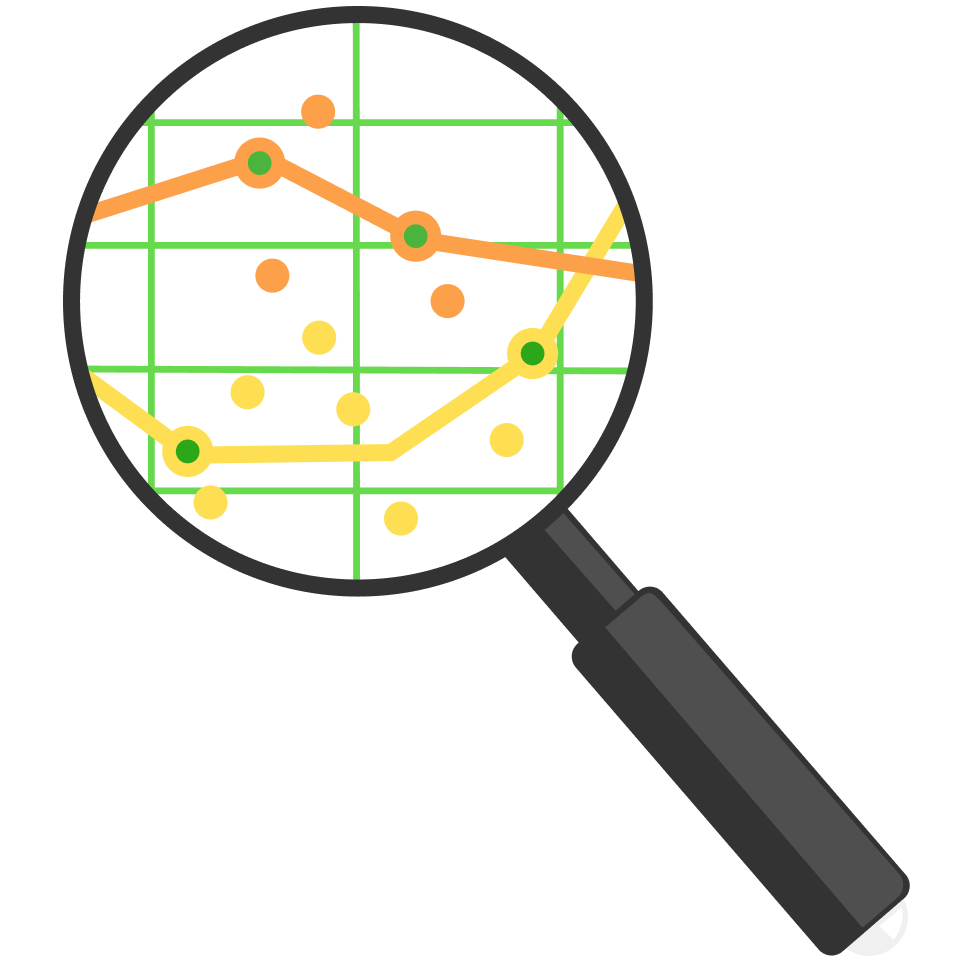# Solving Forwards, Solving Backwards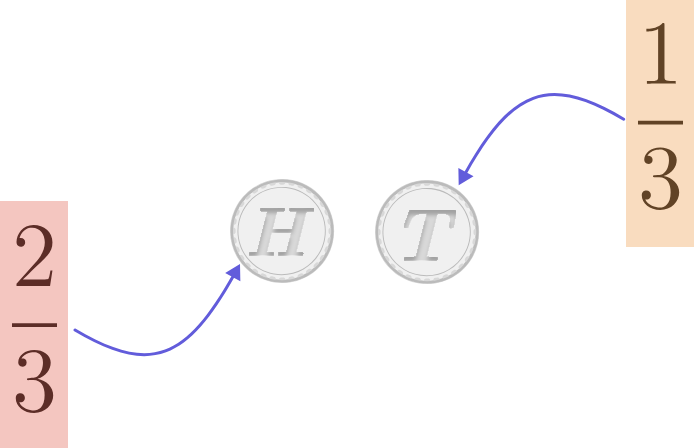Maja has an unfair coin which is weighted so that, when flipped, it has a $$\frac{2}{3}$$ chance of landing on heads and $$\frac{1}{3}$$ chance of landing on tails.

If she flips the coin twice in a row, what is the probability it shows the same side both times?

# Solving Forwards, Solving Backwards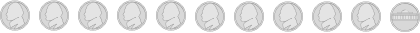Maja has a second coin which she thinks is unfair, like her other coin, but she isn't sure. She'd like to test the coin out by flipping it multiple times.

How many times does she need to flip the coin to be 100% certain of if the coin is fair?

# Solving Forwards, Solving Backwards

Probability is a forwards problem. It starts with a well-defined rule (like two-thirds of coin flips coming up heads) and then asks a question about the data that can be laid out in an exact way. While the result of an actual test may differ, we can exactly quantify what should happen.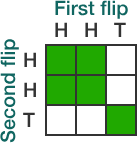Statistics is a backwards problem. It starts from data and then asks what was used to generate it. For example, we might look at a series of 20 coin flips and try to determine if, given 15 heads and 5 tails, the coin might be fair, and if the coin is unfair, to what extent?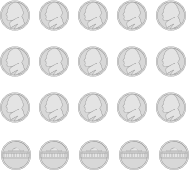Starting from the end result and looking backwards can lead to many possible origin points. Nonetheless, with statistics we can mathematically quantify predictions, and to be a responsible statistician we also must quantify our uncertainty.

Even given these conditions, statistics can help find truths about data in astounding ways.

# Solving Forwards, Solving BackwardsAny statistical prediction should generally have some amount of error. We might try to count the fish in 3 lakes by random sampling, and determine:Suppose we want calculate the cumulative fish count across all three ponds. The estimate would be $$55 + 41 + 32 = 128.$$ What would the accumulated possible error be?

# Solving Forwards, Solving Backwards

Note in the previous problem we still have uncertainty as to our maximum and minimum. If we insisted on a range that covered 100% of possible errors that range is potentially unlimited, so we pick some threshold (assuming, for example, if the same result occurred in 1000 universes, it occurs by luck only in 3 of them).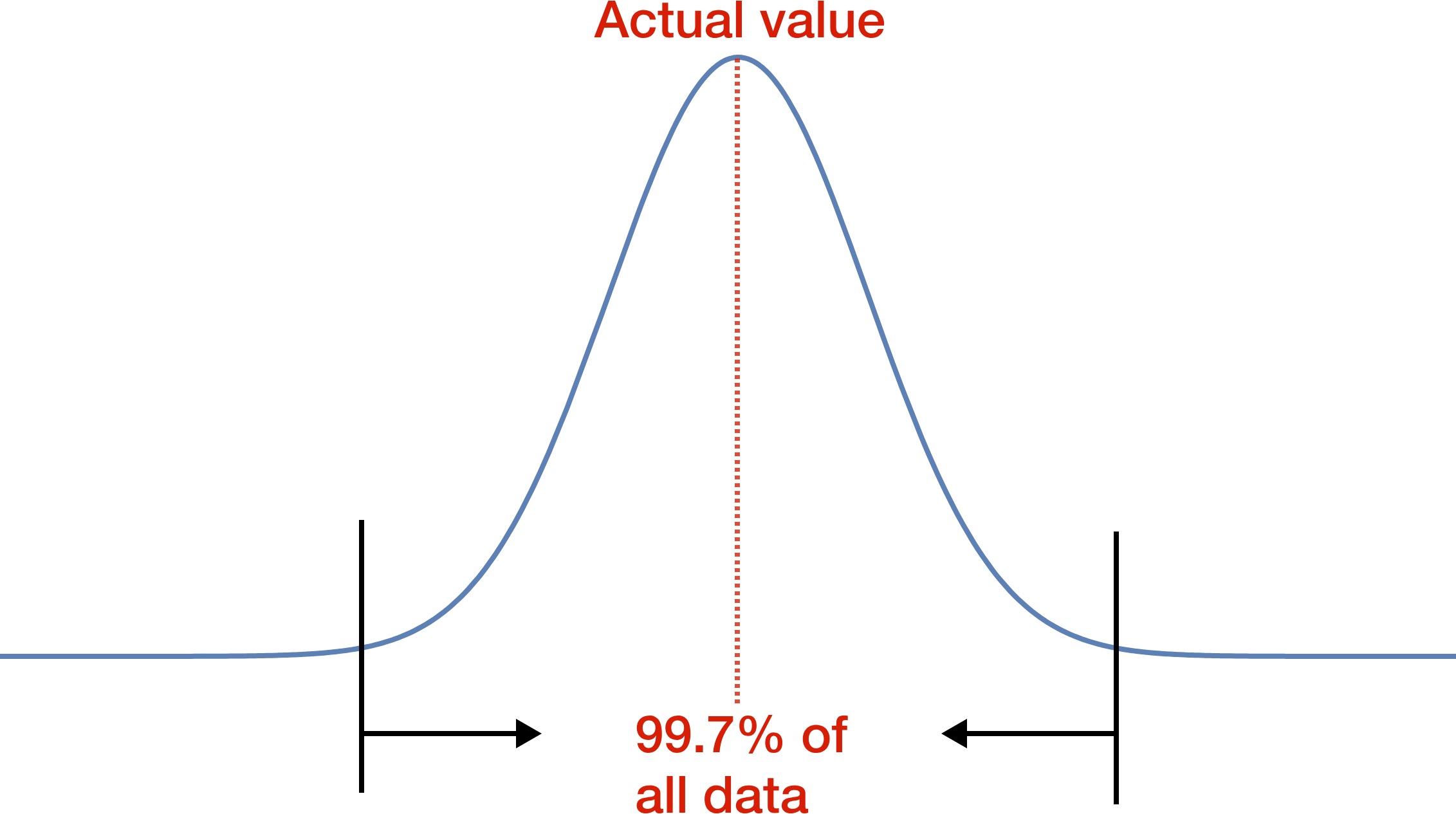One tool for setting the threshold is assuming multiple experiments would form a normal curve (or bell curve), as shown above. (The height of the curve at a particular point indicates proportionally how many observations will fall there.) Assuming the normal curve applies, 99.7% of all observations would fall within the range marked.

Which of the two curves below is marked correctly? (The 99.7% marks are left for clarity.)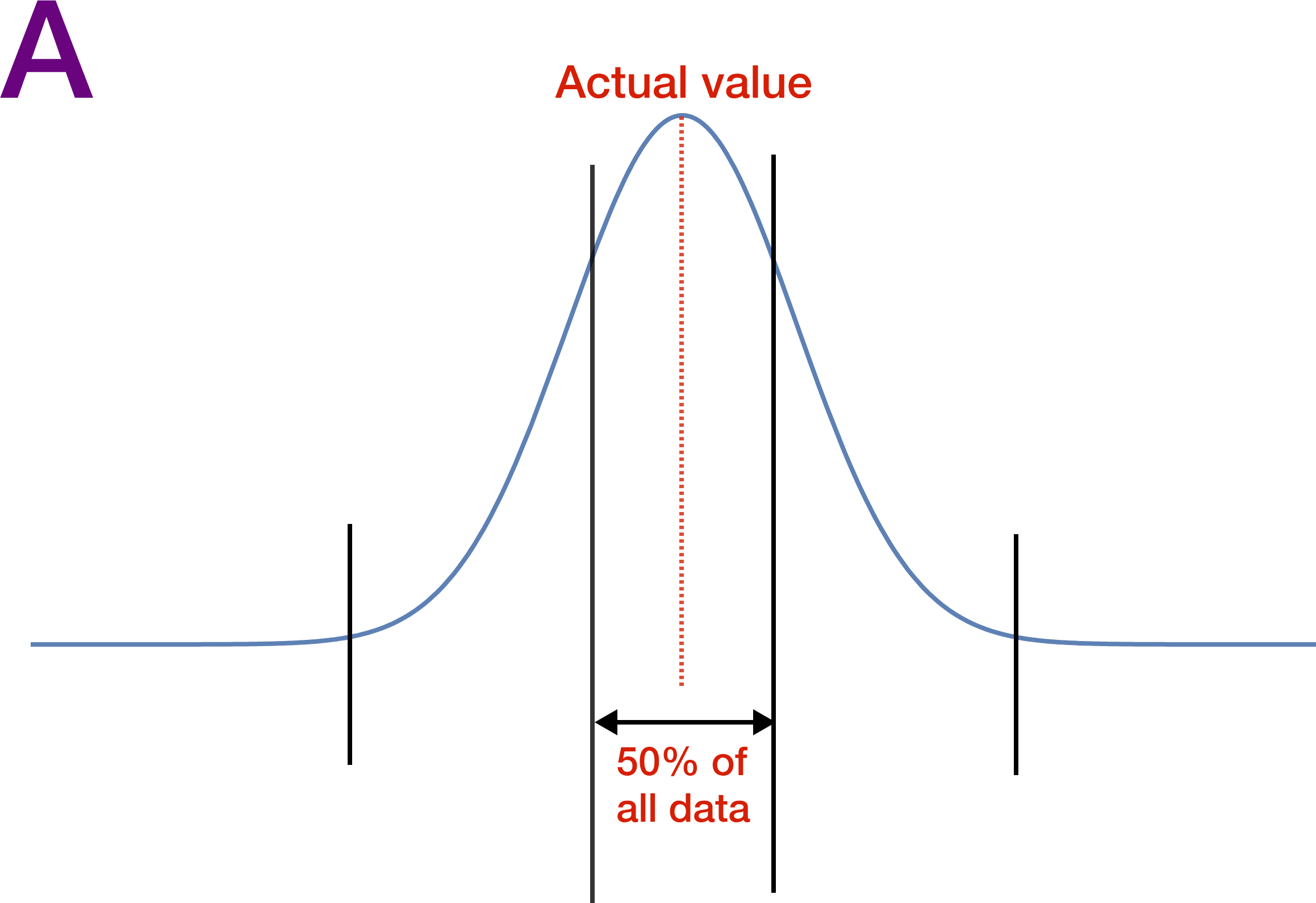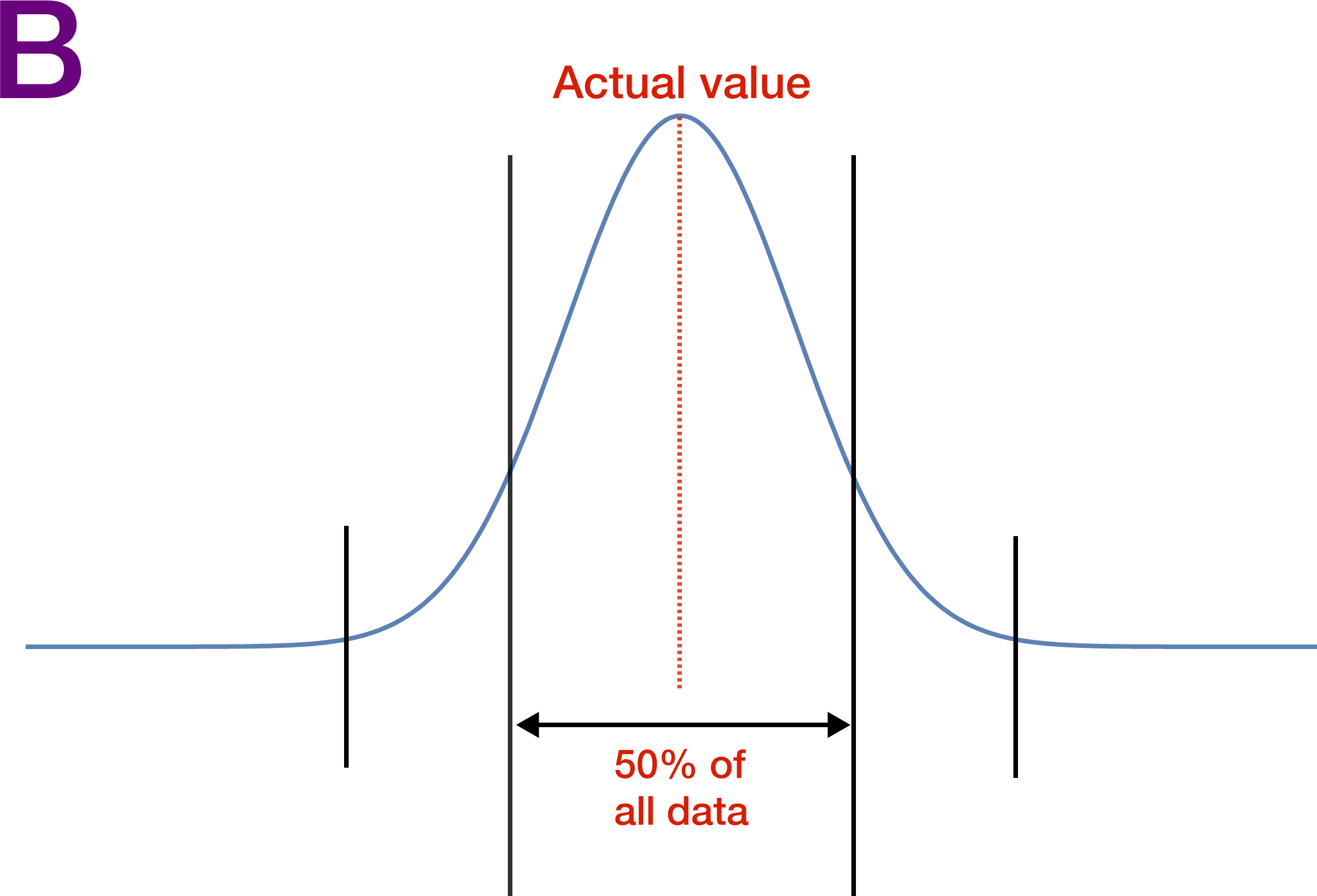# Solving Forwards, Solving Backwards

Let's step back and think on a smaller scale than the previous problem, but still in terms of a threshold where we feel some data is unlikely.Maja thinks her coin is unfair. She flips it 4 times and gets heads every time. She calculates that this would only occur with a fair coin roughly 6% of the time. Should she conclude there is a roughly 94% chance that her coin is unfair?

# Solving Forwards, Solving Backwards

In case you didn't check the answer to the previous problem, here's some more intuition. Reduce the problem to just two coin flips that both come up heads. A fair coin will only do this 25% of the time.However, it seems absurd to claim this means there is a 75% chance the coin is fake! We can put a threshold on when we start to think data looks suspicious, but it is dependent on both judgment and context, and the threshold is not the same as the probability our conclusion is correct.

There are circumstances where data with a 5% chance of occurring is deemed significant, and there are times where the cutoff should be around 0.00006%. This is part of what makes backwards problems both so difficult and so intriguing.

Move on to the next quiz to explore some ways statistics can deceive us, both intentionally and unintentionally.

×